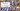# Convert Roman to Integer number - Leet Code Solution

September 13, 2019## Problem Statement

Roman numerals are represented by seven different symbols: I, V, X, L, C, D and M.

``````Symbol       Value
I             1
V             5
X             10
L             50
C             100
D             500
M             1000``````

For example, two is written as II in Roman numeral, just two one’s added together. Twelve is written as, XII, which is simply X + II. The number twenty seven is written as XXVII, which is XX + V + II.

Roman numerals are usually written largest to smallest from left to right. However, the numeral for four is not IIII. Instead, the number four is written as IV. Because the one is before the five we subtract it making four. The same principle applies to the number nine, which is written as IX. There are six instances where subtraction is used:

``````I can be placed before V (5) and X (10) to make 4 and 9.
X can be placed before L (50) and C (100) to make 40 and 90.
C can be placed before D (500) and M (1000) to make 400 and 900.``````

Given a roman numeral, convert it to an integer. Input is guaranteed to be within the range from 1 to 3999.

## Solution

Its a rather easy solution. You need to maintain a mapping. The extra condition here is, the input character can be of one or two char long.

So, you need to fetch if there is a mapping exist for 2 characters and 1 character both.

``````public int romanToInt(String s) {
Map<String, Integer> map = new HashMap();
map.put("I", 1); map.put("V", 5); map.put("X", 10);
map.put("L", 50); map.put("C", 100); map.put("D", 500); map.put("M", 1000);

map.put("IV", 4); map.put("IX", 9); map.put("XL", 40); map.put("XC", 90);
map.put("CD", 400); map.put("CM", 900);

int num = 0;
int l = s.length();
for (int i=0; i<l; ) {
String single = new String(new char[]{s.charAt(i)});
String two = null;
if (i+1 < l) {
two = new String(new char[]{s.charAt(i), s.charAt(i+1)});
}

if (two != null && map.get(two) != null) {
num += map.get(two);
i += 2;
}
else {
num += map.get(single);
i++;
}
}

return num;
}``````